Next: Is there a signal? Up: Poisson model: dependence on Previous: Combination of results: general   Contents

## Including systematic effects

A last interesting case is when there are systematic errors of unknown size in the detector performance. Independently of where systematic errors may enter, the final result will be an uncertainty on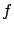. In the most general case, the uncertainty can be described by a probability density function: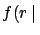best knowledge on experiment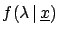For simplicity we analyse here only the case of a single experiment. In the case of many experiments, we only need to iterate the Bayesian inference, as has often been shown in these notes.

Following the general lines given in Section, the problem can be solved by considering the conditional probability, obtaining :Data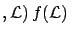Data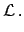d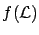(9.11)

The case of absolutely precise knowledge ofis recovered when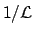is a Dirac delta.

Let us treat in some more detail the case of null observation (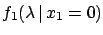). For each possible value ofone has an exponential of expected value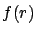[see Eq. ()]. Each of the exponentials is weighted with. This means that, ifis rather symmetrical around its barycentre (expected value), in a first approximation the more and less steep exponentials will compensate, and the result of integral () will be close to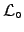calculated in the barycentre of, i.e. in its nominal value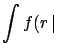:Data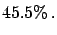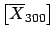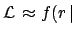Datad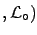Data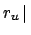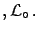Data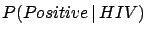Data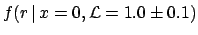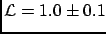To make a numerical example, let us consider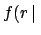(arbitrary units), withfollowing a normal distribution. The upper plot of Fig.shows the difference between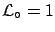Datacalculated applying Eq. () and the result obtained with the nominal value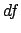: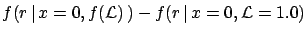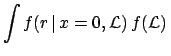(9.12)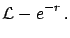d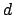(9.13)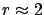is negative up to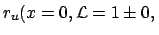, indicating that systematic errors normally distributed tend to increase the upper limit. But the size of the effect is very tiny, and depends on the probability level chosen for the upper limit. This can be seen better in the lower plot of Fig., which shows the integral of the difference of the two functions. The maximum difference is for. As far as the upper limits are concerned, we obtain (the large number of -- non-significatant--digits is only to observe the behaviour in detail):at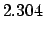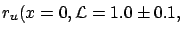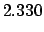atat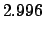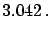at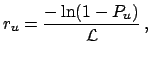An uncertainty of 10% due to systematics produces only a 1% variation of the limits.

To simplify the calculation (and also to get a feeling of what is going on) we can use some approximations.

1. Since the dependence of the upper limit of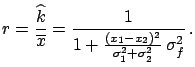fromis given bythe upper limit averaged with the belief onis given by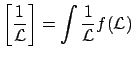E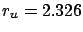dWe need to solve an integral simpler than in the previous case. For the above example ofwe obtain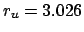at 90% and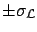at 95%.
2. Finally, as a real rough approximation, we can take into account the small asymmetry of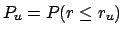around the value obtained at the nominal value ofaveraging the two values ofat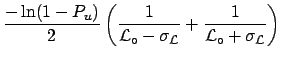from: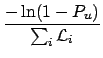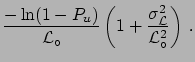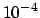We obtain numerically identical results to the previous approximation.
The main conclusion is that the uncertainty due to systematics plays only a second-order role, and it can be neglected for all practical purposes. A second observation is that this uncertainty increases slightly the limits ifis distributed normally, but the effect could also be negative if theis asymmetric with positive skewness.

As a more general remark, one should not forget that the upper limit has the meaning of an uncertainty and not of a value of quantity. Therefore, as nobody really cares about an uncertainty of 10 or 20% on the uncertainty, the same is true for upper/lower limits. At the per cent level it is mere numerology (I have calculated it at the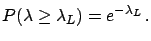level just for mathematical curiosity).Next: Is there a signal? Up: Poisson model: dependence on Previous: Combination of results: general   Contents
Giulio D'Agostini 2003-05-15